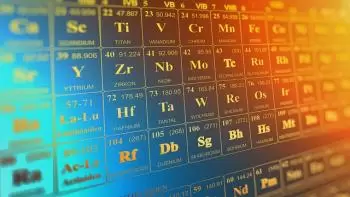# What Are the Requirements for a Nuclear Fusion Reaction?

To obtain a nuclear fusion reaction the atoms involved must overcome an important barrier of electrostatic forces. Due to electrostatic forces two nuclei without electrons repel each other. This repulsion is caused by protons that have a positive charge. However, if two nuclei can get close enough, electrostatic repulsion can be overcome by the quantum effect in which the nuclei can tunnel through the coulomb forces.When a nucleon such as a proton or neutron is added to a nucleus, the nuclear force attracts it to all other nucleons in the nucleus (if the atom is small enough), but mainly to its immediate neighbors due to the short range of force. Nucleons inside a nucleus have more neighboring nucleons than those on the surface.

Smaller cores have a larger surface / volume ratio. Because of this, the binding energy per nucleon due to nuclear force generally increases with the size of the nucleus, but approximates a limit value corresponding to that of a nucleus with a diameter of approximately four nucleons.

## Electrostatic Force

Electrostatic force, on the other hand, is an inverse square force, so a proton added to a nucleus will feel an electrostatic repulsion of all other protons in the nucleus. The electrostatic energy per nucleon due to electrostatic force thus increases without limit as the atomic number of the nucleus grows.

The net result of the opposite electrostatic and strong nuclear forces is that the binding energy per nucleon generally increases with increasing size and then decreases for heavier nuclei. Finally, the binding energy becomes negative and very heavy nuclei are not stable.

An exception to this general trend is the helium-4 nucleus. The binding energy of helium-4 is greater than that of lithium, the next heaviest element. This is because protons and neutrons are fermions. Fermions, according to Pauli's exclusion principle, cannot exist in the same nucleus in exactly the same state. The energy state of each proton or neutron in a nucleus can accommodate both a rotating particle and a rotating particle.

Helium-4 has an abnormally large binding energy because its nucleus consists of two protons and two neutrons, so its four nucleons can be in the fundamental state. Any additional nucleon would have to enter higher energy states. In fact, the helium-4 nucleus is so tightly bound that it is commonly treated as a quantum mechanical particle in nuclear physics, namely the alpha particle.

The situation is similar if two cores are joined. As they get closer, all protons in one nucleus repel all protons in the other. It is not until the two nuclei get close enough that the strong nuclear force can take over (by way of a tunnel) that the repulsive electrostatic force is exceeded. Consequently, even when the final energy state is lower, there is a great energy barrier that must first be overcome. It is called the Coulomb barrier.

The Coulomb barrier is the smallest for hydrogen isotopes, since their nuclei contain a single positive charge. A diproton is not stable, so neutrons must also be involved, ideally so that a helium core, with its extremely tight junction, is one of the products.

The cross section of the reaction (σ) is a measure of the probability of a fusion reaction as a function of the relative velocity of the two reactive nuclei. If the reagents have a velocity distribution, for example, a thermal distribution, then it is useful to average the product cross-sectional distributions and velocity. This average is called 'reactivity', denoted σv. The reaction rate (mergers per volume per time) is σv times the product of the reagent number densities.

## Deuterium Tritium Fuel

Deuterium is one of the two stable isotopes of hydrogen. The nucleus of a deuterium atom, called deuteron, contains a proton and a neutron, while the much more common protium has no neutrons in the nucleus.

Tritium is a rare and radioactive isotope of hydrogen. The tritium nucleus contains a proton and two neutrons, while the common hydrogen-1 isotope nucleus contains only one proton, and the hydrogen-2 nucleus (deuterium) contains a proton and a neutron.

Using deuterium-tritium fuel, the resulting energy barrier is approximately 0.1 MeV. In comparison, the energy needed to remove an electron from hydrogen is 13.6 eV, approximately 7500 times less energy. The (intermediate) result of the fusion is an unstable 5 He nucleus, which immediately expels a neutron with 14.1 MeV. The recoil energy of the remaining 4 He core is 3.5 MeV, so the total energy released is 17.6 MeV. This is many times more than what was needed to overcome the energy barrier.

The melt reaction rate increases rapidly with temperature until it is maximized and then gradually decreases. The DT rate peaks at a lower temperature (approximately 70 keV, or 800 million kelvin) and at a higher value than other reactions commonly considered for fusion energy.

Author:

Published: January 15, 2020
Last review: January 15, 2020